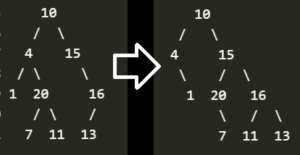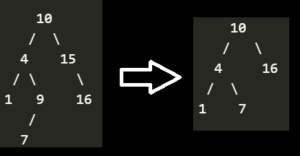# Josh Technology Group Interview Experience for SD (On-Campus)

• Difficulty Level : Medium
• Last Updated : 15 Oct, 2020

Online Selection Test: This comprised of two phases. Both of which were on the same day.

• Phase 1 (Online Objective round): Set of 50 simple MCQs on C++, aptitude, and logical. Only those who cleared the objective round were allowed to sit for the subjective round.
• Phase 2 (Online Subjective round): In this round, we had to write the whole program along with appropriate comments. The code should contain explanations wherever required. Many students who wrote the correct code and passed all test cases were not shortlisted because they forgot to write the comments. You could even include some edge cases in the comments and how they are being handled.

Technical Rounds: The majority of questions were asked on trees and linked lists. (and a little bit of DP). There was a total of 3 technical rounds followed by an HR Round. In each technical round, we were asked 2 questions. Sometimes if the candidate is unable to come up with the solution to any of the problems then another question is added (depends on the interviewer’s mood). Technical round 1 and 2 held on the same day while 3rd round was after a week.

Technical Round 1:

Technical Round 2:

1. Maximum sum of elements in array such that no two elements are adjacent to each other.
2. Re-arrange the linked list such that first node=firstnode-last node, second node=second node-2nd last node.
Example:

```Input : 1 -> 2 -> 3 -> 5 -> 9 -> 6 -> 3 -> 2 -> 0
Output: 1-> 0 -> 0 -> -1 -> 9 -> 6 -> 3 -> 2 -> 0
Explanation: (1-0)->(2-2)->(3-3)->(5-6)->9->6->3->2->0```
3. Shift all the nodes to the right if there is an empty place in a Binary tree. Every level should be filled from the rightmost side. See image for example:Technical Round 3:

1. Minimum no. of jumps required to reach the other side of the bridge. The bridge is represented in the form of an array consisting of 1s and 0s. 1 represents a valid step while 0 represents a broken step. We are given another array which consists of no of steps we can cover in one jump. We need to find the minimum jumps required to reach another end.
Example:
`Bridge=[1,0,0,1,1,1,0,1,1,0,0,0,1,1,1,0,1] Jumps=[2,3,5,6] Output: 4 `

Explanation: initially we are at the 0th index. We can take a jump of a maximum 5 (a jump of 6 lands us on 0). From the 5th index we take a jump of 3 and reach on index 8, From 8 we take a jump of 6 and land on 14. From 14 we take a final jump of 2 and reach the other end. We reached the other end in 4 jumps.

2. Given a BST we need to check if all the root nodes value is the average of its left and right node. If the condition is false then delete the root node. Return a BST that follows the above condition.3. A recent project that you worked on.

HR Round: